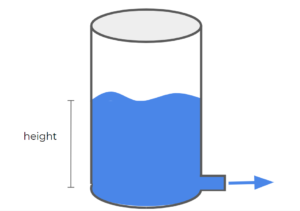# 39 Introduction to Unsteady-state Operations

Learning Objectives

By the end of this section, you should be able to:

Derive material balance equations

Unsteady-State: in unsteady-state processes, mass and energy in the system are changing with time (not constant).

Understanding and modeling these systems allow us to:

• Control system variables by adjusting parameters
• Predict the system’s behavior during start-up and shutdown

Example: Draining Tank

Consider the following draining tank:This tank demonstrates a very simple unsteady-state system. Taking the tank as our system of interest, as the water drains from the tank, the system’s overall mass changes. The height of the liquid in the tank also changes as the liquid drains out of the tank.

## General Balance Equation

To characterize systems (generally process units), we can do a balance on any quantity (total mass, energy, moles, etc.). For this class, we will focus on mass, energy, and mole balances, but we could do these balances on any quantity.

$Input - Output + Generation - Consumption = Accumulation$

• Input: Enters through the system boundaries ($IN$)
• Output: Exits through the system boundaries ($OUT$)
• Generation: Produced in the system ($GEN$)
• Consumption: Consumed in the system ($CON$)
• Accumulation: Build-up or overall loss in the system ($ACC$)

Note that the generation and consumption terms are often associated with chemical reactions

$IN - OUT + GEN - CON = ACC$

Exercise: Balance Terms

Consider a reactor with continuous inlet and exit streams. A reaction occurs in the reactor that is first order:

$A → B$

The inlet stream only contains species A and the reaction goes to completion in the reactor. The reactor is initially filled entirely with A. What terms in the following expression are considered (i.e. non-zero) for this system when assessing the moles of species A as the reaction starts occurring?

$IN - OUT + GEN - CON = ACC$

### Solution

Since the reaction goes to completion in the reactor, there is no A exiting in the outlet stream. Therefore, $OUT$ will be omitted from the expression. This reaction is a non-reversible reaction, so no A is generated in the system ( $GEN=0$). All the other terms are present, A is fed in ( $IN$), A is consumed or reacted ( $CON$) and the amount of A in the reactor will change with time as B is produced ( $ACC$).

$IN-CON=ACC$

Because we start with a reactor filled with A initially, the moles of A decreases in the reactor during start-up, and approaches a steady-state, as shown in the image below. The change in moles of A in the reactor represents the ACC term in the balance.

## Transient Balances

Transient balances are any balances that where some quantities involved in the balance are functions with time. If we have a continuous process, we can analyze what happens to a given quantity for a given change in time $\Delta t$. As an example, let’s take the mass of a given species (we’ll call species A) to be our quantity of interest:

$IN - OUT + GEN - CON = ACC$

$\dot{m}_{in,A}*\Delta t - \dot{m}_{out,A}*\Delta t + \dot{r}_{gen,A}*\Delta t - \dot{r}_{con,A}*\Delta t = \Delta M_{A}$

Where:

• $\dot{m}_{in,A}$, $\dot{m}_{out,A}$ are the mass flows in and out of the system of species A
• $\dot{r}_{gen,A}$, $\dot{r}_{con,A}$ are the rates of generation and consumption of A in the system
• $M_{A}$ is the total mass of A in the system at a given time

If we want to turn this into a differential balance, we will isolate $\Delta t$ and let it approach to 0 (just like in calculus classes):

$\dot{m}_{in,A}*\Delta t - \dot{m}_{out,A}*\Delta t + \dot{r}_{gen,A}*\Delta t - \dot{r}_{con,A}*\Delta t = \Delta M_{A}$

$(\dot{m}_{in,A} - \dot{m}_{out,A} + \dot{r}_{gen,A} - \dot{r}_{con,A})*\Delta t = \Delta M_{A}$

$(\dot{m}_{in,A} - \dot{m}_{out,A} + \dot{r}_{gen,A} - \dot{r}_{con,A}) = \frac{\Delta M_{A}}{\Delta t}$

$(\dot{m}_{in,A} - \dot{m}_{out,A} + \dot{r}_{gen,A} - \dot{r}_{con,A}) = \frac{dM_{A}}{dt}$

We can mutiply $dt$ on both sides, and take the integral of each term to calculate the change of each term over time:

$\dot{m}_{in,A}dt - \dot{m}_{out,A}dt + \dot{r}_{gen,A}dt - \dot{r}_{con,A}dt = dM_{A}$

$\int_{t_i}^{t_f}\dot{m}_{in,A}dt - \int_{t_i}^{t_f}\dot{m}_{out,A}dt + \int_{t_i}^{t_f}\dot{r}_{gen,A}dt - \int_{t_i}^{t_f}\dot{r}_{con,A}dt = \int_{M_i}^{M_f}dM_{A}$

For steady-state processes, the $\frac{dM_{A}}{dt}$ term goes to 0, as there would be no change in the total mass of A in the system over time. For unsteady-state processes, this differential equation must be solved using boundary conditions.

An example of a boundary condition is $M_{A}(t=0)= M_i$

This equations says that the mass of A in the system at time (t = 0) is equal to $M_i$. This mass can be equal to 0, meaning that there is no A in our system at time zero. It can also be equal to a specific value, meaning that our system starts with an initial mass of A.

### Differential Balances General Procedure

1. Omit any terms that are equal to zero or negligible from the mass, energy, or mole balances.
2. Write an expression for the total amount of the quantity of interest (mass, energy, moles) using the remaining terms in the balance
3. Determine the initial conditions of the system and solve accordingly